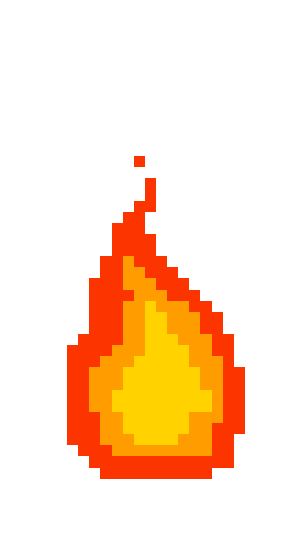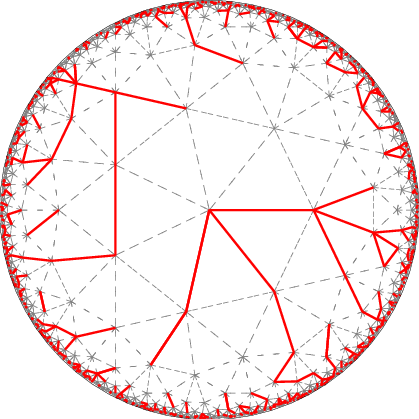# link..linkPoint Curry DiscoVisual Search Engine!# Is your friend full of it?

Posted on Sat 02 September 2017 in misc

## Five Thirty Eight Riddler Express Problem - Sep 1, 2017¶

You’re hanging out with some friends, shooting the breeze and talking sports. One of them brags to the group that he once made 17 free throws in a row after years of not having touched a basketball. You think the claim sounds unlikely, but plausible. Another friend scoffs, thinking it completely impossible. Let’s give your bragging friend the benefit of the doubt and say he’s a 70-percent free-throw shooter.

So, who’s right? What is the number of free throws that a 70-percent shooter would be expected to take before having a streak of 17 makes in a row? And what if his accuracy was a bit worse?

This looks like a pretty innocuous Bernoulli's trials problem but it isn't! Had the question been about the average number of throws taken for 17 successes, the answer would have been straightforward - $$0.7\sum_{n=16}^{\infty} n\binom {n} {16}0.7^{16}0.3^{n-16} = 17/0.7 \approx 24.3$$

But the question we are dealing with is about the average number of throws that are required for 17 consecutive makes in a row. I have a vague recollection of this problem being dealt with in Feller's Volume 1 and it not being pretty at all.

I fooled around a bit and tried to count sequences of the kind $S_20S_1$, where $S_1$ is a sequence of 17 ones and $S_2$ is a sequence of zeroes and ones of any length such that $S_2$ does not contain $S_1$ as a substring but gave up after seeing how this approach quickly degenerated into an absolute mess. Maybe a recursive way of generating $S_2$ sequences could do the trick.

# Simulation Time!¶

At first, I thought I should replicate the entire process as it is, by doing runs of shooting the ball till 17 consecutive throws are successful and calculate the average number of attempts. For example, each run can be mapped to a string comprising only s and f characters, representing success and failure respectively, with one character generated at a time (with their respective probabilities), that terminates when s turns up 17 times consecutively. Repeat this a million times and calculate the average length of all these strings and we have the answer. This would involve setting up a counter for the number of times s is generated consecutively and resetting the counter to zero when an $f$ is generated and repeating it till s appears 17 times consecutively for each run.

It then occurred to me that since these are all independent trials, I could just generate one long string of say 100 million characters, find all occurences of $S_1=sssssssssssssssss$ substrings in that and treat every substring between two adjacent $S_1$ substrings as a successful run(sans the success tail)! I must admit I am pretty pleased with myself here.

In :
import numpy as np
import re

def averageNumberOfThrows(accuracy):
y = np.random.choice(('s', 'f'), 100000000, p=[accuracy, 1-accuracy]).tostring()
seventeenShots = 's'*17

a = [m.start() for m in re.finditer(seventeenShots, y)]
b = [a[index+1]-value for index, value in enumerate(a) if index < len(a) - 1]

return sum([a + 17] + b)/(len(b) + 1)

In :
averageNumberOfThrows(0.7)

Out:
1424

# How this varies with the shooter's accuracy¶

In :
import plotly.plotly as py
import plotly.graph_objs as go

data = [averageNumberOfThrows(accuracy) for accuracy in np.arange(0.6,0.71,0.01)]

pata = [go.Scatter(
x=np.arange(0.6,0.71,0.01),
y=data,
mode='lines+markers',
line=dict(shape='spline')
)]

py.iplot(pata, filename='spline-interpolation')

Out:

Quite a lot it seems! Average number of attempts goes all the way upto 14000 if the accuracy goes down to 60%

In :
averageNumberOfThrows(0.8)

Out:
189
In :
averageNumberOfThrows(0.9)

Out:
44